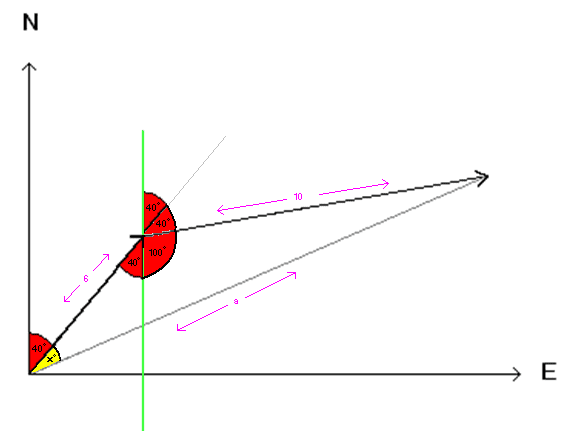• Kaldanis

## Homework Statement

If the wind drives a yacht at 080° with a speed of 10 knots and the tide is running at 6 knots at 040°, what is the actual velocity of the yacht?

## The Attempt at a Solution

I started by drawing the two vectors (6 and 10) head to tail. I want to find the length of the resultant vector (side a) and angle x° (the yellow one). So first, I drew the green line parallel to the north line which allowed me to fill in the angles around it. I'm now completely stuck on how to figure out side a and angle x°. If anyone could point me in the right direction I'd be greateful!The x component of the resultant a is the sum of the x components of vectors 6 and 10. Likewise for the y component of a. So, can you find the x and y components of vectors 6 and 10?

let the velocity due to wind is a (vector)
let the velocity due to waves is b (vector)
let the net velocity is c (vector)

find a and b in form of x(i) + y(j) + z(k)

Then use triangle law of addition: c = a + b